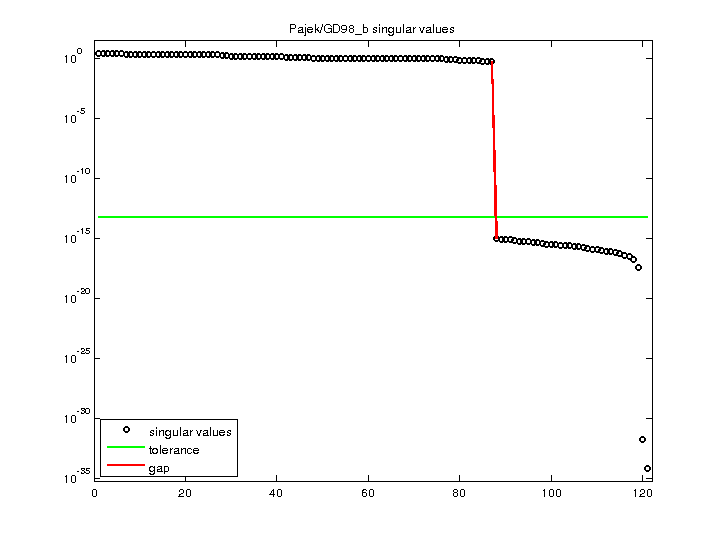Matrix: Pajek/GD98_b

Description: Pajek network: Graph Drawing contest 1998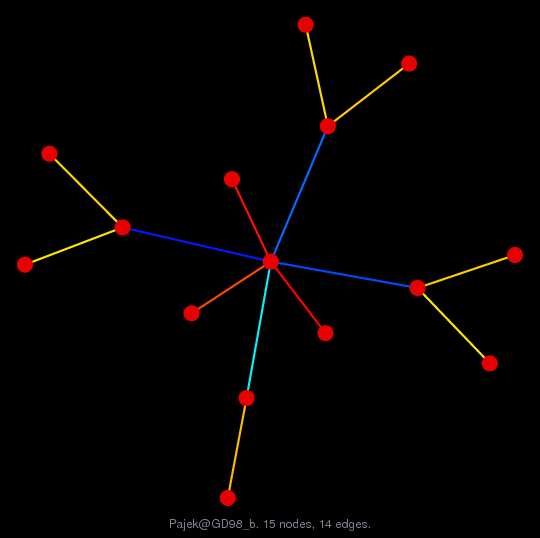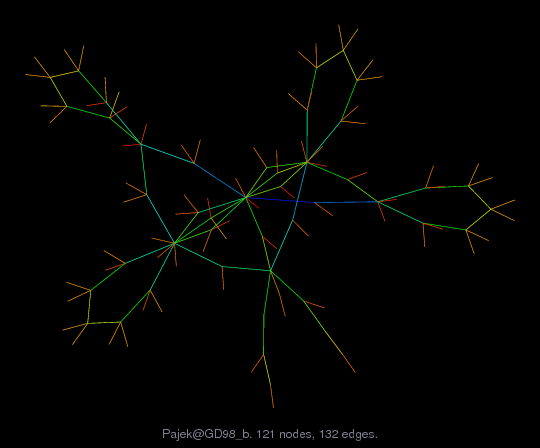(bipartite graph drawing) (graph drawing of A+A')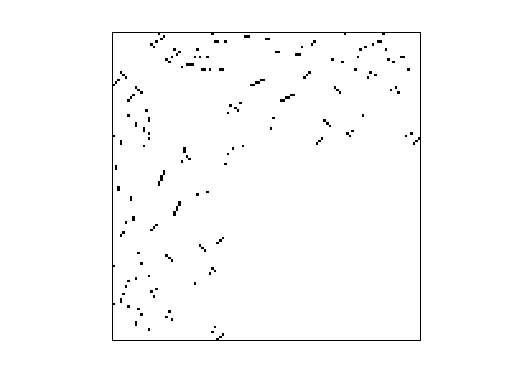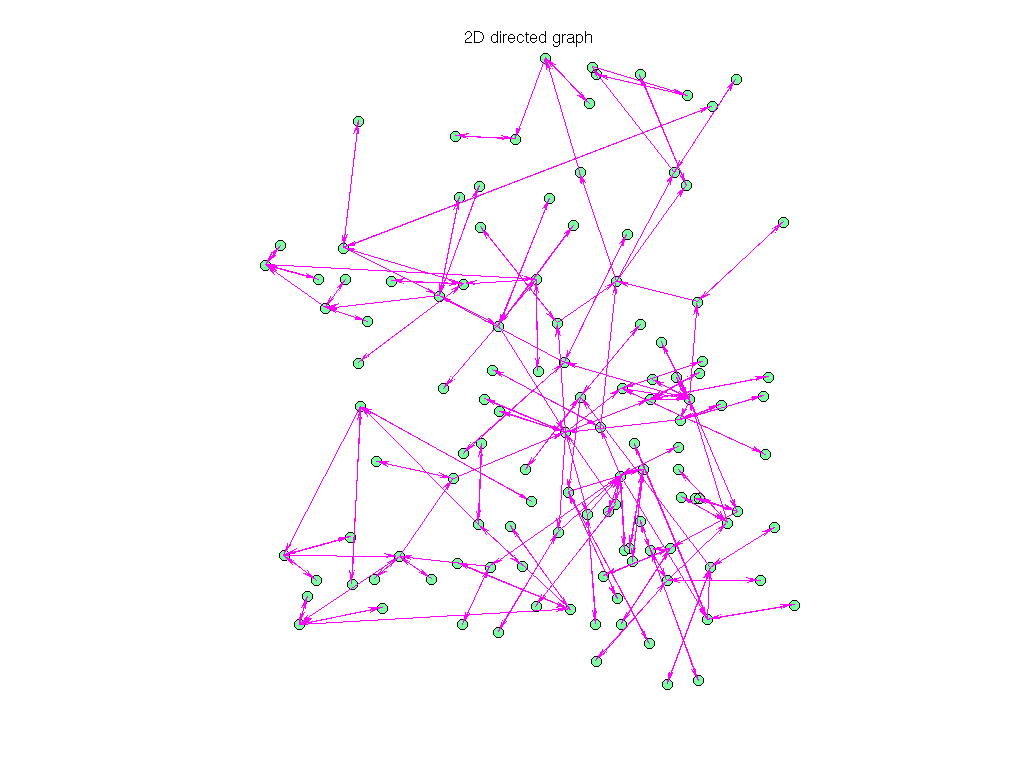• Matrix group: Pajek
• download as a MATLAB mat-file, file size: 3 KB. Use UFget(1496) or UFget('Pajek/GD98_b') in MATLAB.

 Matrix properties number of rows 121 number of columns 121 nonzeros 207 # strongly connected comp. 12 explicit zero entries 0 nonzero pattern symmetry 72% numeric value symmetry 72% type binary structure unsymmetric Cholesky candidate? no positive definite? no

 author Graph Drawing Contest editor V. Batagelj date 1998 kind directed graph 2D/3D problem? no

 Additional fields size and type nodename full 121-by-6 coord full 121-by-2

Notes:

```------------------------------------------------------------------------------
Pajek network converted to sparse adjacency matrix for inclusion in UF sparse
matrix collection, Tim Davis.  For Pajek datasets, See V. Batagelj & A. Mrvar,
------------------------------------------------------------------------------
The original problem had 3D xyz coordinates, but all values of z were equal
to 0.5241, and have been removed.  This graph has 2D coordinates.
```

 SVD-based statistics: norm(A) 2.84969 min(svd(A)) 6.32821e-35 cond(A) 4.50314e+34 rank(A) 87 null space dimension 34 full numerical rank? no singular value gap 5.27174e+14

 singular values (MAT file): click here SVD method used: s = svd (full (A)) ; status: ok